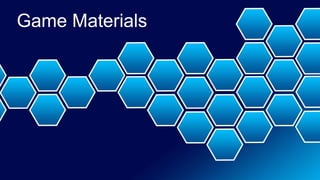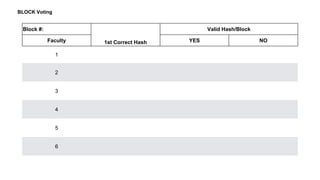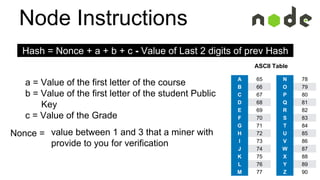Seu SlideShare está sendo baixado. ×

# Blockchain game for kids.pptx

Anúncio
Anúncio
Anúncio
Anúncio
Anúncio
Anúncio
Anúncio
Anúncio
Anúncio
Anúncio
AnúncioCarregando em…3
×

1 de 41 Anúncio

# Blockchain game for kids.pptx

A Blockchain (or block chain) is a method of storing a list of entries, which cannot be changed easily after they are created. This also applies to the list. This is done by using several concepts from cryptography, including digital signatures and hash functions. This presentation is the materials to run a blockchain game with students. Through gameplay, students can better understand what blockchain is, how it is being used, and ways they can develop ideas around this technology.

A Blockchain (or block chain) is a method of storing a list of entries, which cannot be changed easily after they are created. This also applies to the list. This is done by using several concepts from cryptography, including digital signatures and hash functions. This presentation is the materials to run a blockchain game with students. Through gameplay, students can better understand what blockchain is, how it is being used, and ways they can develop ideas around this technology.

Anúncio
Anúncio

### Blockchain game for kids.pptx

1. 1. Game Materials
2. 2. Block #: 1st Correct Hash Valid Hash/Block Faculty YES NO 1 2 3 4 5 6 BLOCK Voting
3. 3. Node Instructions N 78 O 79 P 80 Q 81 R 82 S 83 T 84 U 85 V 86 W 87 X 88 Y 89 Z 90 A 65 B 66 C 67 D 68 E 69 F 70 G 71 H 72 I 73 J 74 K 75 L 76 M 77 ASCII Table a = Value of the first letter of the course b = Value of the first letter of the student Public Key c = Value of the Grade value between 1 and 3 that a miner with provide to you for verification Hash = Nonce + a + b + c - Value of Last 2 digits of prev Hash Nonce =
4. 4. Node Instructions N 78 O 79 P 80 Q 81 R 82 S 83 T 84 U 85 V 86 W 87 X 88 Y 89 Z 90 A 65 B 66 C 67 D 68 E 69 F 70 G 71 H 72 I 73 J 74 K 75 L 76 M 77 ASCII Table a = Value of the first letter of the course b = Value of the first letter of the student Public Key c = Value of the Grade value between 1 and 3 that a miner with provide to you for verification Hash = Nonce + a + b + c - Value of Last 2 digits of prev Hash Nonce =
5. 5. Node Instructions N 78 O 79 P 80 Q 81 R 82 S 83 T 84 U 85 V 86 W 87 X 88 Y 89 Z 90 A 65 B 66 C 67 D 68 E 69 F 70 G 71 H 72 I 73 J 74 K 75 L 76 M 77 ASCII Table a = Value of the first letter of the course b = Value of the first letter of the student Public Key c = Value of the Grade value between 1 and 3 that a miner with provide to you for verification Hash = Nonce + a + b + c - Value of Last 2 digits of prev Hash Nonce =
6. 6. Miner Instructions N 78 O 79 P 80 Q 81 R 82 S 83 T 84 U 85 V 86 W 87 X 88 Y 89 Z 90 A 65 B 66 C 67 D 68 E 69 F 70 G 71 H 72 I 73 J 74 K 75 L 76 M 77 Lookup Table a = Value of the first letter of the course b = Value of the first letter of the student Public Key c = Value of the Grade value between 1 and 3 that you will adjust to calculate a hash that can be equally divisible by 3 Nonce = Hash = Nonce + a + b + c - Value of Last 2 digits of prev Hash
7. 7. Miner Worksheet Nouse + a + b + c - Value of Last 2 digits of prev Hash = Hash Divide by 3 Remainder 1 + + + - = 2 + + + - = 3 + + + - =
8. 8. Miner Worksheet Nouse + a + b + c - Value of Last 2 digits of prev Hash = Hash Divide by 3 Remainder 1 + + + - = 2 + + + - = 3 + + + - =
9. 9. Miner Worksheet Nouse + a + b + c - Value of Last 2 digits of prev Hash = Hash Divide by 3 Remainder 1 + + + - = 2 + + + - = 3 + + + - =
10. 10. Miner Worksheet Nouse + a + b + c - Value of Last 2 digits of prev Hash = Hash Divide by 3 Remainder 1 + + + - = 2 + + + - = 3 + + + - =
11. 11. Miner Worksheet Nouse + a + b + c - Value of Last 2 digits of prev Hash = Hash Divide by 3 Remainder 1 + + + - = 2 + + + - = 3 + + + - =
12. 12. Miner Worksheet Nouse + a + b + c - Value of Last 2 digits of prev Hash = Hash Divide by 3 Remainder 1 + + + - = 2 + + + - = 3 + + + - =
13. 13. Miner Worksheet Nouse + a + b + c - Value of Last 2 digits of prev Hash = Hash Divide by 3 Remainder 1 + + + - = 2 + + + - = 3 + + + - =
14. 14. Miner Instructions N 78 O 79 P 80 Q 81 R 82 S 83 T 84 U 85 V 86 W 87 X 88 Y 89 Z 90 A 65 B 66 C 67 D 68 E 69 F 70 G 71 H 72 I 73 J 74 K 75 L 76 M 77 Lookup Table a = Value of the first letter of the course b = Value of the first letter of the student Public Key c = Value of the Grade value between 1 and 3 that you will adjust to calculate a hash that can be equally divisible by 3 Nonce = Hash = Nonce + a + b + c - Value of Last 2 digits of prev Hash
15. 15. Miner Worksheet Nouse + a + b + c - Value of Last 2 digits of prev Hash = Hash Divide by 3 Remainder 1 + + + - = 2 + + + - = 3 + + + - =
16. 16. Miner Worksheet Nouse + a + b + c - Value of Last 2 digits of prev Hash = Hash Divide by 3 Remainder 1 + + + - = 2 + + + - = 3 + + + - =
17. 17. Miner Worksheet Nouse + a + b + c - Value of Last 2 digits of prev Hash = Hash Divide by 3 Remainder 1 + + + - = 2 + + + - = 3 + + + - =
18. 18. Miner Worksheet Nouse + a + b + c - Value of Last 2 digits of prev Hash = Hash Divide by 3 Remainder 1 + + + - = 2 + + + - = 3 + + + - =
19. 19. Miner Worksheet Nouse + a + b + c - Value of Last 2 digits of prev Hash = Hash Divide by 3 Remainder 1 + + + - = 2 + + + - = 3 + + + - =
20. 20. Miner Worksheet Nouse + a + b + c - Value of Last 2 digits of prev Hash = Hash Divide by 3 Remainder 1 + + + - = 2 + + + - = 3 + + + - =
21. 21. Miner Worksheet Nouse + a + b + c - Value of Last 2 digits of prev Hash = Hash Divide by 3 Remainder 1 + + + - = 2 + + + - = 3 + + + - =
22. 22. Miner Instructions N 78 O 79 P 80 Q 81 R 82 S 83 T 84 U 85 V 86 W 87 X 88 Y 89 Z 90 A 65 B 66 C 67 D 68 E 69 F 70 G 71 H 72 I 73 J 74 K 75 L 76 M 77 Lookup Table a = Value of the first letter of the course b = Value of the first letter of the student Public Key c = Value of the Grade value between 1 and 3 that you will adjust to calculate a hash that can be equally divisible by 3 Nonce = Hash = Nonce + a + b + c - Value of Last 2 digits of prev Hash
23. 23. Miner Worksheet Nouse + a + b + c - Value of Last 2 digits of prev Hash = Hash Divide by 3 Remainder 1 + + + - = 2 + + + - = 3 + + + - =
24. 24. Miner Worksheet Nouse + a + b + c - Value of Last 2 digits of prev Hash = Hash Divide by 3 Remainder 1 + + + - = 2 + + + - = 3 + + + - =
25. 25. Miner Worksheet Nouse + a + b + c - Value of Last 2 digits of prev Hash = Hash Divide by 3 Remainder 1 + + + - = 2 + + + - = 3 + + + - =
26. 26. Miner Worksheet Nouse + a + b + c - Value of Last 2 digits of prev Hash = Hash Divide by 3 Remainder 1 + + + - = 2 + + + - = 3 + + + - =
27. 27. Miner Worksheet Nouse + a + b + c - Value of Last 2 digits of prev Hash = Hash Divide by 3 Remainder 1 + + + - = 2 + + + - = 3 + + + - =
28. 28. Miner Worksheet Nouse + a + b + c - Value of Last 2 digits of prev Hash = Hash Divide by 3 Remainder 1 + + + - = 2 + + + - = 3 + + + - =
29. 29. Miner Worksheet Nouse + a + b + c - Value of Last 2 digits of prev Hash = Hash Divide by 3 Remainder 1 + + + - = 2 + + + - = 3 + + + - =
31. 31. Below is your key pair for the grade blockchain. Your teacher will assign a grade to your public key. You can then use any of the grade scanning tools to review the blockchain and retrieve your grades. 2 Student Public Key Private Key bd9ebc 4382af3398
32. 32. Below is your key pair for the grade blockchain. Your teacher will assign a grade to your public key. You can then use any of the grade scanning tools to review the blockchain and retrieve your grades. 3 Student Public Key Private Key da603d 187235b94a
33. 33. Below is your key pair for the grade blockchain. Your teacher will assign a grade to your public key. You can then use any of the grade scanning tools to review the blockchain and retrieve your grades. 4 Student Public Key Private Key fc45e2 39c2ef1cb6
34. 34. Below is your key pair for the grade blockchain. Your teacher will assign a grade to your public key. You can then use any of the grade scanning tools to review the blockchain and retrieve your grades. 5 Student Public Key Private Key c67445 56164d905c
35. 35. Below is your key pair for the grade blockchain. Your teacher will assign a grade to your public key. You can then use any of the grade scanning tools to review the blockchain and retrieve your grades. 6 Student Public Key Private Key bde7af f674f230d4
36. 36. Below is your key pair for the grade blockchain. Your teacher will assign a grade to your public key. You can then use any of the grade scanning tools to review the blockchain and retrieve your grades. 7 Student Public Key Private Key e2dd8a ae4710fff0
37. 37. Unused Slides
38. 38. Rogue Miner Instructions N 78 O 79 P 80 Q 81 R 82 S 83 T 84 U 85 V 86 W 87 X 88 Y 89 Z 90 A 65 B 66 C 67 D 68 E 69 F 70 G 71 H 72 I 73 J 74 K 75 L 76 M 77 ASCII Table a = Value of the first letter of the course b = Value of the first letter of the student Public Key c = Value of the Grade value between 1 and 6 that you will adjust to calculate a hash that can be equally divisible by 2 AND 3 Hash = Nonce + a + b + c - Value of Last 2 digits of prev Hash Nonce =
39. 39. Miner Instructions N 78 O 79 P 80 Q 81 R 82 S 83 T 84 U 85 V 86 W 87 X 88 Y 89 Z 90 A 65 B 66 C 67 D 68 E 69 F 70 G 71 H 72 I 73 J 74 K 75 L 76 M 77 Table a = Value of the first letter of the course b = Value of the first letter of the student Public Key c = Value of the Grade value between 1 and 6 that you will adjust to calculate a hash that can be equally divisible by 2 AND 3 Nonce = Hash = Nonce + a + b + c - Value of Last 2 digits of prev Hash
40. 40. Miner Worksheet Nouse + a + b + c - Value of Last 2 digits of prev Hash = Hash Divide by 2 Remainder Divide by 3 Remainder 1 + + + - = 2 + + + - = 3 + + + - = 4 + + + - = 5 + + + - = 6 + + + - =
41. 41. Rogue Miner Worksheet Nouse + a + b + c - Value of Last 2 digits of prev Hash = Hash Divide by 2 Remainder Divide by 3 Remainder 1 + + + - = 2 + + + - = 3 + + + - = 4 + + + - = 5 + + + - = 6 + + + - =

### Notas do Editor

• Note: Print as many of these as blocks you want to process
Note the Faculty # who correctly Mines the Hash
When you get to 4 Faculty (Nodes) that agree on the correct hash then that is over 51% and the block is added.